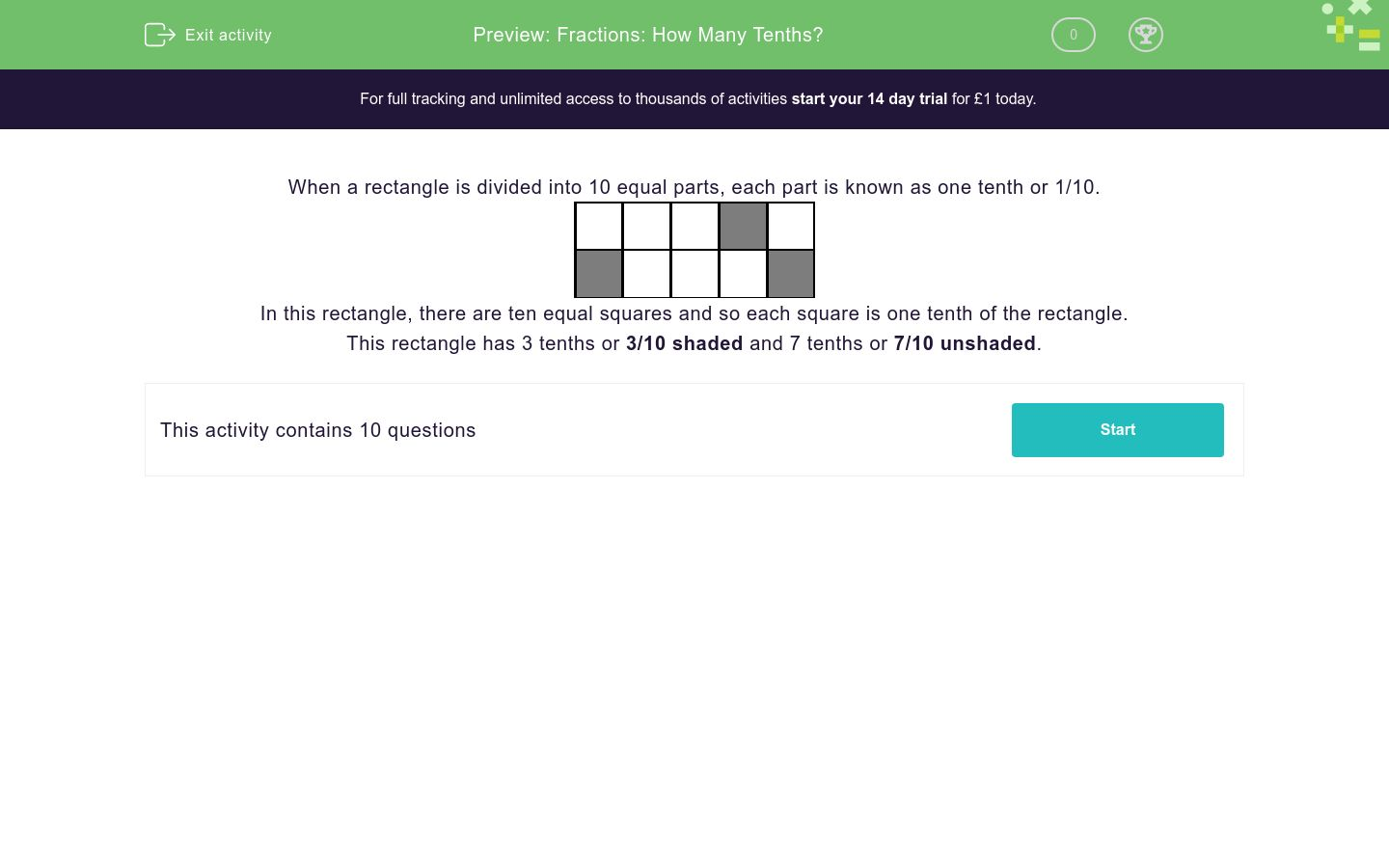# Fractions: How Many Tenths?

In this worksheet, students work out how many tenths of a shape have been shaded.Key stage:  KS 2

Curriculum topic:   Number: Fractions

Curriculum subtopic:   Count in Tenths

Difficulty level:### QUESTION 1 of 10

When a rectangle is divided into 10 equal parts, each part is known as one tenth or 1/10.In this rectangle, there are ten equal squares and so each square is one tenth of the rectangle.

This rectangle has 3 tenths or 3/10 shaded and 7 tenths or 7/10 unshaded.

---- OR ----